Courses

# Speed of Sound (Part - 1) Civil Engineering (CE) Notes | EduRev

## Civil Engineering (CE) : Speed of Sound (Part - 1) Civil Engineering (CE) Notes | EduRev

The document Speed of Sound (Part - 1) Civil Engineering (CE) Notes | EduRev is a part of the Civil Engineering (CE) Course Fluid Mechanics - Notes, Videos, MCQs & PPTs.
All you need of Civil Engineering (CE) at this link: Civil Engineering (CE)

Speed of Sound

• The so-called sound speed is the rate of propogation of a pressure pulse of infinitesimal strength through a still fluid. It is a thermodynamic property of a fluid.
• A pressure pulse in an incompressible flow behaves like that in a rigid body. A displaced particle displaces all the particles in the medium. In a compressible fluid, on the other hand, displaced mass compresses and increases the density of neighbouring mass which in turn increases density of the adjoining mass and so on. Thus, a disturbance in the form of an elastic wave or a pressure wave travels through the medium. If the amplitude and theerfore the strength of the elastic wave is infinitesimal, it is termed as acoustic wave or sound wave.
• Figure 39.1(a) shows an infinitesimal pressure pulse propagating at a speed " a " towards still fluid (= 0) at the left. The fluid properties ahead of the wave are p,T and p while the properties behind the wave are p+dp, T+dT and  p +dp. The fluid velocity dV is directed toward the left following wave but much slower.
• In order to make the analysis steady, we superimpose a velocity " a " directed towards right, on the entire system (Fig. 39.1(b)). The wave is now stationary and the fluid appears to have velocity " a " on the left and (a - dV) on the right. The flow in Fig. 39.1 (b) is now steady and one dimensional across the wave. Consider an area A on the wave front. A mass balance gives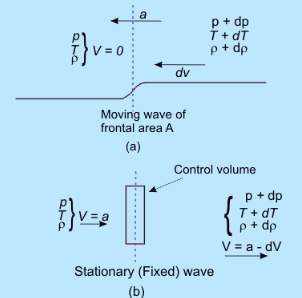Fig 39.1: Propagation of a sound wave
(a) Wave Propagating into still Fluid        (b) Stationary Wave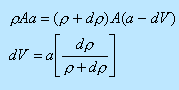(39.1)

This shows that

(a) dv = 0 if dρ is positive.

(b) A compression wave leaves behind a fluid moving in the direction of the wave (Fig. 39.1(a)).

(c) Equation (39.1) also signifies that the fluid velocity on the right is much smaller than the wave speed " a ". Within the framework of infinitesimal strength of the wave (sound wave), this " a " itself is very small.

• Applying the momentum balance on the same control volume in Fig. 39.1 (b). It says that the net force in the x direction on the control volume equals the rate of outflow of x momentum minus the rate of inflow of x momentum. In symbolic form, this yields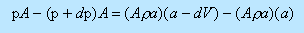In the above expression, Aρa is the mass flow rate. The first term on the right hand side represents the rate of outflow of x-momentum and the second term represents the rate of inflow of x momentum.

• Simplifying the momentum equation, we get

If the wave strength is very small, the pressure change is small.

Combining Eqs (39.1) and (39.2), we get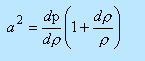(39.3a)

he larger the strength dp/p  of the wave ,the faster the wave speed; i.e., powerful explosion waves move much faster than sound waves.In the limit of infinitesimally small strength,  dp → 0  we can write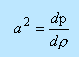(39.3b)

Note that

(a) In the limit of infinitesimally strength of sound wave, there are no velocity gradients on either side of the wave. Therefore, the frictional effects (irreversible) are confined to the interior of the wave.

(b) Moreover, the entire process of sound wave propagation is adiabatic because there is no temperature gradient except inside the wave itself.

(c) So, for sound waves, we can see that the process is reversible adiabatic or isentropic.

So the correct expression for the sound speed is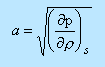(39.4)

For a perfect gas, by using of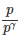= const and p = pRT, we deduce the speed of sound as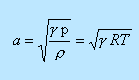(39.5)

For air at sea-level and at a temperature of 150C, a=340 m/s

Pressure Field Due to a Moving Source

• Consider a point source emanating infinitesimal pressure disturbances in a still fluid, in which the speed of sound is "a". If the point disturbance, is stationary then the wave fronts are concentric spheres. As shown in Fig. 39.2(a), wave fronts are present at intervals of Δt .

• Now suppose that source moves to the left at speed U < a. Figure 39.2(b) shows four locations of the source, 1 to 4, at equal intervals of time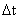, with point 4 being the current location of the source.

• at point 1, the source emanated a wave which has spherically expanded to a radius 3aΔt  in an interval of time 3Δt During this time the source has moved to the location 4 at a distance of 3uΔt   from point 1. The figure also shows the locations of the wave fronts emitted while the source was at points 2 and 3, respectively.

• When the source speed is supersonic U > a (Fig. 39.2(c)), the point source is ahead of the disturbance and an observer in the downstream location is unaware of the approaching source. The disturbance emitted at different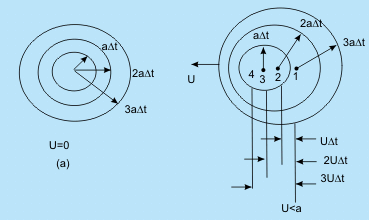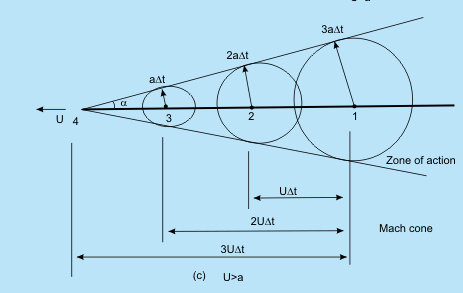Fig 39.2 Wave fronts emitted from a point source in a still fluid when the source speed is
(a) U = 0 (still Source)    (b) U < a (Subsonic)   (c) U > a (Supersonic)

points of time are enveloped by an imaginary conical surface known as "Mach Cone"The half angle of the cone α is known as Mach angle and given by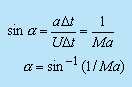Since the disturbances are confined to the cone, the area within the cone is known as zone of action and the area outside the cone is zone of silence

An observer does not feel the effects of the moving source till the Mach Cone covers his position.

Basic Equations for One-Dimensional Flow

• Here we will study a class of compressible flows that can be treated as one dimensional flow. Such a simplification is meaningful for flow through ducts where the centreline of the ducts does not have a large curvature and the cross-section of the ducts does not vary abruptly.

• In one dimension, the flow can be studied by ignoring the variation of velocity and other properties across the normal direction of the flow. However, these distributions are taken care of by assigning an average value over the cross-section (Fig. 39.3).

• The area of the duct is taken as A(x) and the flow properties are taken as p(x), ρ(x), V(x) etc. The forms of the basic equations in a one-dimensional compressible flow are;

• Continuity Equation
• Energy Equation
• Bernoulli and Euler Equations
• Momentum Principle for a Control Volume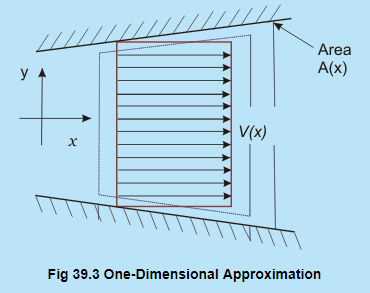Fig 39.3 One-Dimensional Approximation

Offer running on EduRev: Apply code STAYHOME200 to get INR 200 off on our premium plan EduRev Infinity!

,

,

,

,

,

,

,

,

,

,

,

,

,

,

,

,

,

,

,

,

,

;